8th Grade Math Home > Teacher Resources > Activities > Two for One Box Company: Student Worksheet

Two for One Box Company: Student Worksheet

Name: ____________________________________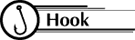Does a box twice as big hold twice as much? (Twice as big here means doubling each dimension)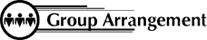Students work individually or in pairs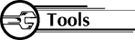• Several sheets of 1 centimeter square grid paper
• Quantity of 1 centimeter cubes for each group
• Handouts for each student
• Scissors
• Tape1. Have students draw a net (a two-dimensional pattern that can be formed to make a solid) for making an open top box on the grid paper for each of the sets of dimensions listed below.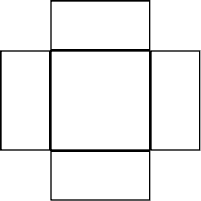Example

2. Cut out the net and fold up each side to form the open box and tape the sides.
3. Find the volume of the box by filing it with 1 centimeter cubes, and record the results in a table such as the one below.

 BOX DIMENSIONS VOLUME A 2 X 2 X 2 B 3 X 3 X 3 C 4 X 4 X 4 D 5 X 5 X 5 E 2 X 4 X 6 F 2 X 3 X 4

4. Write a ratio between the first dimension of Box A and Box B.
5. Write a ratio between the volumes of Box A and Box B.
6. Are they equivalent?
7. If not, what can you do to the ratio of dimensions to make it equivalent to the ratio of volumes?
8. How would you change the dimensions of a box to ensure that the volume is doubled?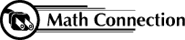As a result of this activity, students will understand how changing dimensions of a figure affects its volume.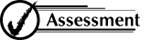Have students find volumes for several different prisms when given the ratio of the dimensions of the prism and the volume of one prism.

 8th Grade Math Home > Teacher Resources > Activities > Two for One Box Company: Student Worksheet